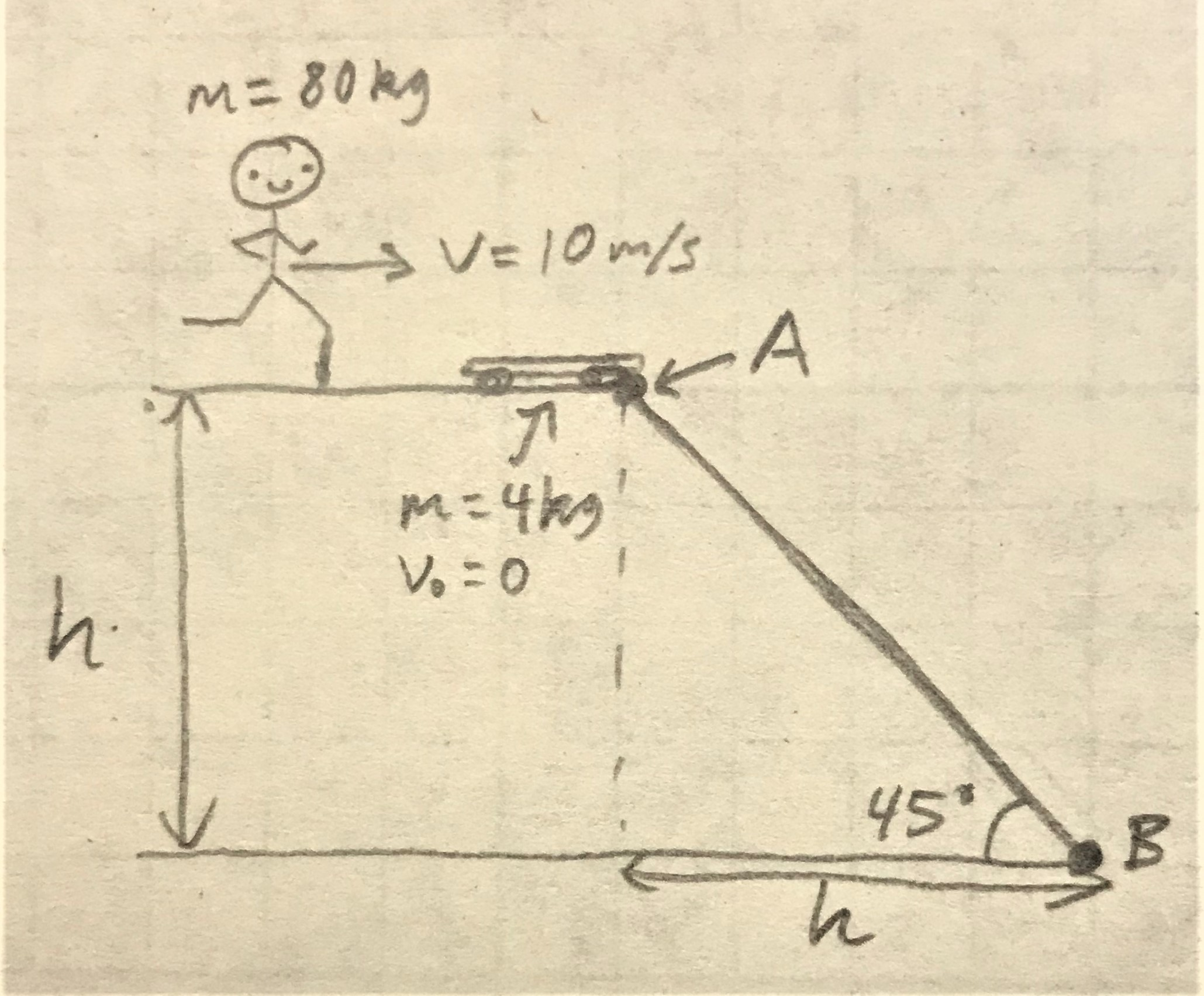Question
17 views

Hi, I don't know if I was given enough or have too much uneccesary information to solve this problem, but here it is.

An 80 kg guy is running on a flat surface at a velocity of 10 m/s.  He gets on a 4 kg skateboard that is sitting at rest at the top of a 45-degree inclined plane (see drawing).  If the time taken to travel from point A to point B is 6 seconds and only gravitational acceleration is acting on the guy/skateboard unit, find

a) the velocity of the unit when t = 3 seconds;

b) the height h at point A.

I'm also not sure which part should be solved first.  Thanks.help_outlineImage TranscriptioncloseM= 80kg M3D VE10 m/s .A m=4kg' V.D0 45 fullscreen
check_circle

Step 1

First, apply momentum conservation, and solve for the velocity of the unit at point A along horizontal direction vH.

Step 2

Now consider the diagram for the velocity and acceleration along the incline, that is the direction of motion from point A to point B. The component of the velocity vH along the incline will be the initial velocity of unit for the motion between the point A and B.

Step 3

(a)

From the diagram, use equation of motion, and solve for the veloc...

### Want to see the full answer?

See Solution

#### Want to see this answer and more?

Solutions are written by subject experts who are available 24/7. Questions are typically answered within 1 hour.*

See Solution
*Response times may vary by subject and question.
Tagged in

### Physics